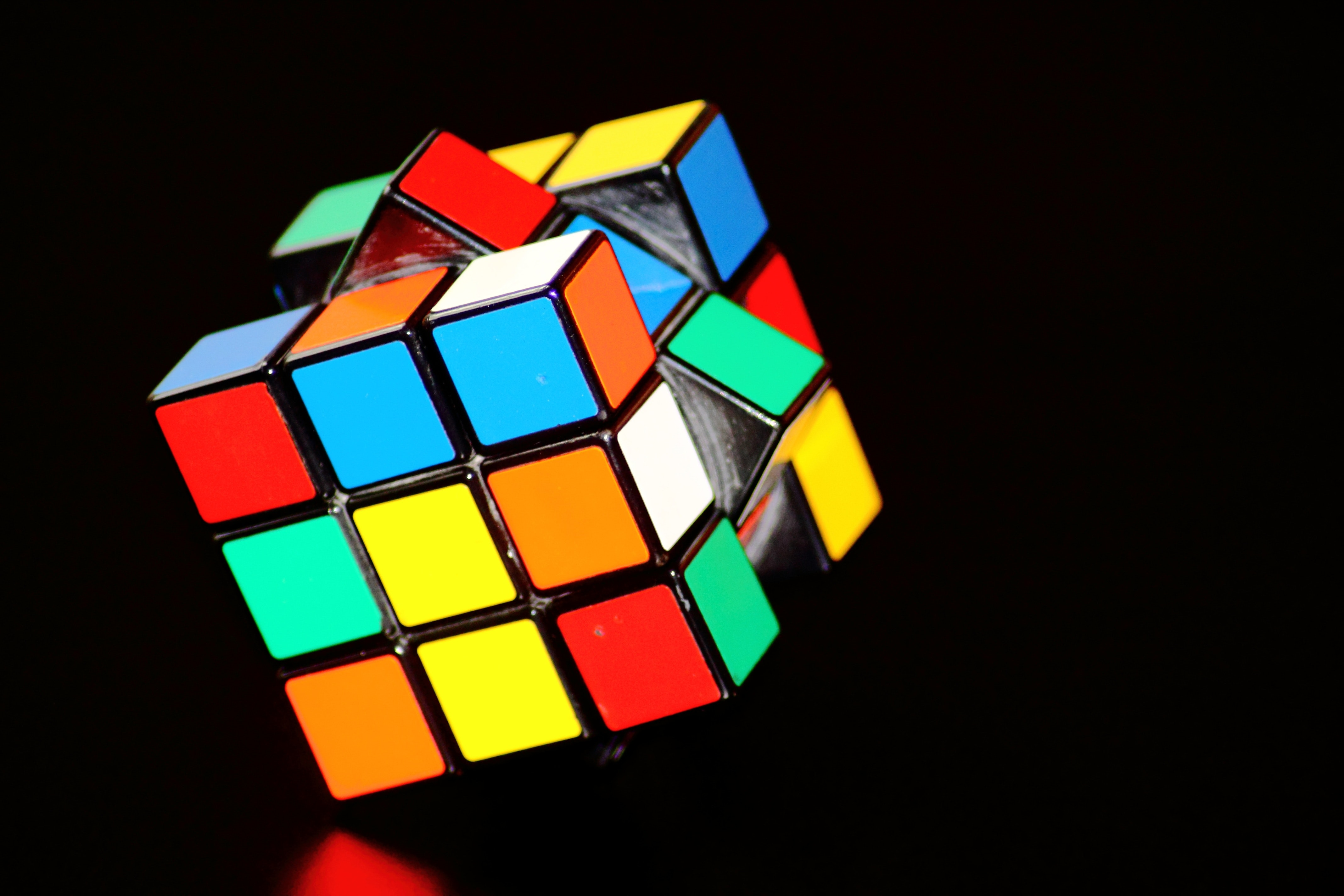# LeetCode Problem 80. Remove Duplicates from Sorted Array II

## Problem

Given a sorted array nums, remove the duplicates in-place such that duplicates appeared at most twice and return the new length.

Do not allocate extra space for another array, you must do this by modifying the input array in-place with O(1) extra memory.

```Example 1:
Given nums = [1,1,1,2,2,3],
Your function should return length = 5, with the first five elements of nums being 1, 1, 2, 2 and 3 respectively.
It doesn't matter what you leave beyond the returned length.```

```Example 2:
Given nums = [0,0,1,1,1,1,2,3,3],
Your function should return length = 7, with the first seven elements of nums being modified to 0, 0, 1, 1, 2, 3 and 3 respectively.
It doesnt matter what values are set beyond the returned length.```

## Solution

`var removeDuplicates = function(nums) {    if(nums.length < 2) {       return nums.length    }        let i = 0    let j = 0        for(let k = 1; k < nums.length; k++) {                // invalid - we already found 2 instances        if(i !== j && nums[k] === nums[j]) {           continue        }                        // apply to i - first instance        if(nums[i] !== nums[k]) {            i = j+1            nums[i] = nums[k]            j = i                        continue        }                        // apply to j - second instance        if(nums[j] === nums[k]) {            j = j+1            nums[j] = nums[k]        }            }        return j+1};`

## Explanation

You have to return `length` of the new array. But, because the array itself is passed by reference the caller knows the new values.

Because we have to sort the array - time complexity would at least be `O(n)`

We can maintain three pointers

• i -> pointing to the first instance of the unique element
• j -> pointing to the second instance of the unique element
• k -> pointing to the current element being inspected

And finally return `j+1`

Relation between `i` and `j` is that they will either be equal or `j` will be 1 place ahead of `i` This constraint will help us make sure that there are at most 2 instances of the given element

As soon as `k` finds the third instance - we will simply continue without doing anything Something like this:

`if(i !== j && nums[k] === nums[j]) {    continue}`

## Sample test cases

```[]-> []
-> 
[1,1,2,2]-> [1,1,2,2]
[1,1,1,2,2,3]-> [1,1,2,2,3]
[1,1,1,2,2,2,2,2,2,2,2,2,2,3]-> [1,1,2,2,3]```

## Highlights

Runtime: 88 ms, faster than 68.14% of JavaScript online submissions for Remove Duplicates from Sorted Array II.

Memory Usage: 38.6 MB, less than 64.88% of JavaScript online submissions for Remove Duplicates from Sorted Array II.

### Disclaimer

This may not be the optimal solution. And that's okay. The purpose here is to practice problem solving and have fun with algorithms. I am constantly learning new optimized solutions for these problems.

Please comment below if you have a better solution. Let's learn algorithms and data structures together.